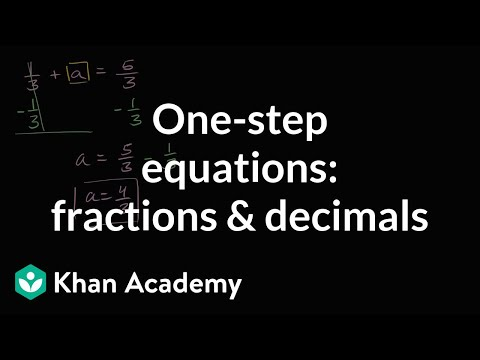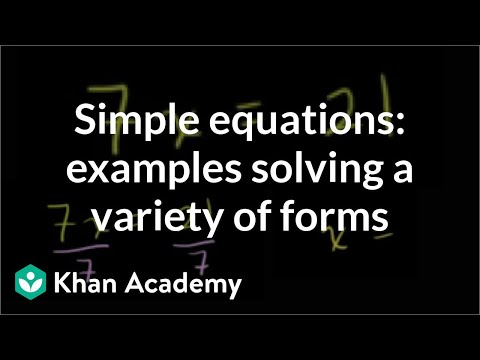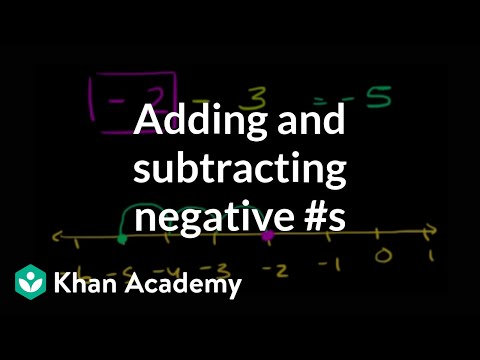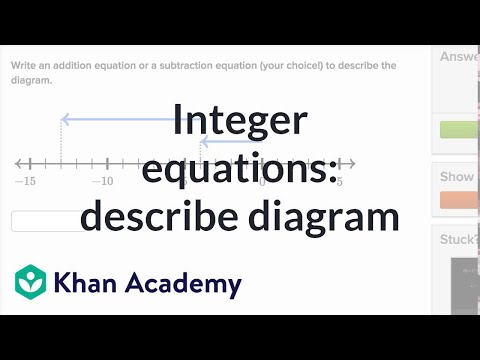# One-step equations with addition and subtraction

##By Khan Academy

Learn how to solve one-step addition and subtraction equations by adding or subtracting the same thing from both sides of the equation.# Solve System of Linear Equations Using Addition Method

##By APUS07

YouTube presents Solve System of Linear Equations Using Addition Method, an educational video resource on math.# Solving One Step Equations By Addition and Subtraction

##By Mathispower4u

Video about solving one step equations by addition and subtraction.# Solving One Step Equations By Addition and Subtraction

##By Mathispower4u

Video about solving one step equations by addition and subtraction.# One-step addition and subtraction equations with fractions and decimals

##By Khan Academy

Learn how to solve one-step addition and subtraction equations by adding or subtracting the same thing from both sides of the equation.# Systems of Linear Equations - Inconsistent Systems Using Elimination by Addition - Example 1

##By PatrickJMT

YouTube presents Systems of Linear Equations - Inconsistent Systems Using Elimination by Addition - Example 1 an educational video resources on math.# One-step addition and subtraction equations with fractions and decimals

##By Khan Academy

Learn how to solve one-step addition and subtraction equations that have fractions and decimals in them.# One-step equations with addition and subtraction

##By Khan Academy

Learn how to solve one-step addition and subtraction equations by adding or subtracting the same thing from both sides of the equation.# One-step equations with addition and subtraction

##By Khan Academy

Some quick examples to practice solving a variety of one step equations. All 4 operations (add, subtract, multiple, divide) are paired with variables.# One-step equations with addition and subtraction

##By Khan Academy

Learn how to solve this equation: a + 5 = 54ï¿½ï¿½ï¿½ï¿½ï¿½ï¿½ï¿½ï¿½ï¿½ï¿½ï¿½ï¿½# Addition elimination method 3 | Systems of equations | 8th grade | Khan Academy

##By Khan Academy

Khan Academy presents Addition Elimination Method 3, an educational video resource on math.# Addition elimination method 2 | Systems of equations | 8th grade | Khan Academy

##By Khan Academy

Khan Academy presents Addition Elimination Method 2, an educational video resource on math.# Addition elimination method 1 | Systems of equations | 8th grade | Khan Academy

##By Khan Academy

Khan Academy presents Addition Elimination Method 1, an educational video resource on math.# Integer addition and subtraction: equations and number lines

##By Khan Academy

Learn how to add and subtract negative numbers. The problems solved in this video are 2 - 3 = -1 and -2 - 3 = -5 and -2 + 3 = 1 and 2 - (-3) = 5 and -2 - (-3).# Integer addition and subtraction: equations and number lines

##By Khan Academy

Integer equations to describe diagram# One-step addition and subtraction equations with fractions and decimals

##By Khan Academy

Some quick examples to practice solving a variety of one step equations. All 4 operations (add, subtract, multiple, divide) are paired with variables.# One-step addition and subtraction equations with fractions and decimals

##By Khan Academy

Learn how to solve this equation: a + 5 = 54ï¿½ï¿½ï¿½ï¿½ï¿½ï¿½ï¿½ï¿½ï¿½ï¿½ï¿½ï¿½# One-step equations with addition and subtraction

##By Khan Academy

Learn how to solve this equation: a + 5 = 54ï¿½ï¿½ï¿½ï¿½ï¿½ï¿½ï¿½ï¿½ï¿½ï¿½ï¿½ï¿½# One-step equations with addition and subtraction

##By Khan Academy

Some quick examples to practice solving a variety of one step equations. All 4 operations (add, subtract, multiple, divide) are paired with variables.# Integer addition and subtraction: equations and number lines

##By Khan Academy

Learn how to add and subtract negative numbers. The problems solved in this video are 2 - 3 = -1 and -2 - 3 = -5 and -2 + 3 = 1 and 2 - (-3) = 5 and -2 - (-3).